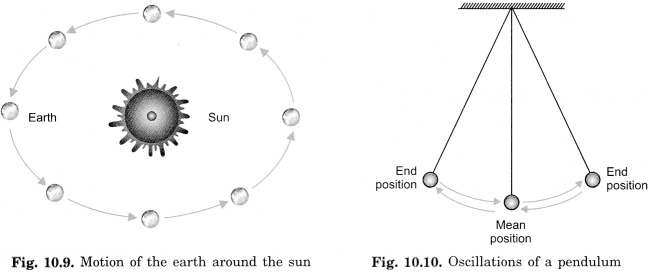# NCERT-6 Science Solutions L-10

## Chapter 10:​​ Motion and Measurement of Distances

NCERT TEXTBOOK EXERCISES-Page 106

Question 1.
Give two examples each, of modes of transport used on land, water, and air.

• Land – Bus, car, bicycle.

• Water – Motor-boat, ship.

• Air – Aeroplane, Helicopter.

Question 2.​​ Fill in the blanks:

• One meter is ………………………………. cm.

• Five​​ kilometres​​ is ………………………………. m.

• The motion of a child on a swing is ………………………………..

• The motion of the needle of a sewing machine is ………………………………..

• The motion of the wheel of a bicycle is ………………………………..

• 100

• 5000

• Periodic (oscillatory) motion

• Periodic motion

• Circular motion

Question 3.​​ Why can a pace or a footstep not be used as a standard unit of length?
A pace or a footstep cannot be used as a standard unit of length because it is different for different persons. Hence correct measurement cannot be made.

Question 4.​​ Arrange the following lengths in their increasing magnitude: 1 meter, 1 centimeter, 1 kilometer, 1 millimeter.
1 millimeter, 1 centimeter, 1 meter, 1 kilometer.

Question 5.​​ The height of a person is 1.65 m. Express it into cm and mm.

(1) 1 m = 100 cm.
​​ 1.65 = 165 cm.

(2) 1 cm = 10 mm
​​ 165 cm = 1650 mm
Hence the height of a person is 165 cm or 1650 mm.

Question 6.​​ The distance between Radha’s home and her school is 3250 m. Express this distance into km.
Given that,

Distance between Radha’s home and her school = 3250 m We have to find out distance in km.

We know that,

1000 m = 1 km

hence, 3250 m =
32501000 km = 3.250 km.

Question 7.​​ While measuring the length of a knitting needle, the reading of the scale at one end is 3.0 cm and at the other end is 33.1 cm. What is the length of the needle?
= 33. cm – 3.0 cm

= 30.1 cm.

Question 8.​​ Write the similarities and differences between the motion of a bicycle and a ceiling fan that has been switched on.

• Similarity: Both the wheels of a bicycle and a ceiling fan execute a circular motion on a fixed axis.

• Dissimilarity: A bicycle also executes a rectilinear motion while a fan does not execute such motion.

Question 9.​​ Why would you not like to use a measuring tape made of an elastic material like rubber to measure distance? What would be some of the problems you would meet in telling someone about a distance you measured with such a tape?
We could not use an elastic measuring tape to measure distance because elastic measuring tape cannot measure correct distance. Because if the elastic measuring tape is stretched more​​ than​​ the distance will be less and if it stretched less than the same distance would be more.
The problems we would meet in​​
talking​​ about the distance we measured with an elastic tape are that the distance would be incorrect and would be different every time measured.

Question 10.​​ Give two examples of periodic motion.